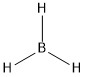# 3.1: Introduction

Symmetry is important in the study of chemistry. It can be studied on both the molecular level and the crystalline level, of which the former will be discussed in this book.

A molecule is symmetrical if it stays in an indistinguishable configuration after some movement. For example, the molecule $$BH_{3}$$ in 3.1 can be rotated about an axis perpendicular to the screen and passes through the boron atom. If the rotation angle is 120°, the new configuration is indistinguishable from the original form.3.1

The study of symmetry has many applications, some of which will be introduced in this chapter. Also in this chapter, some of the terminology and definitions used in group theory, the mathematical representation of symmetry, will be introduced.